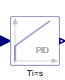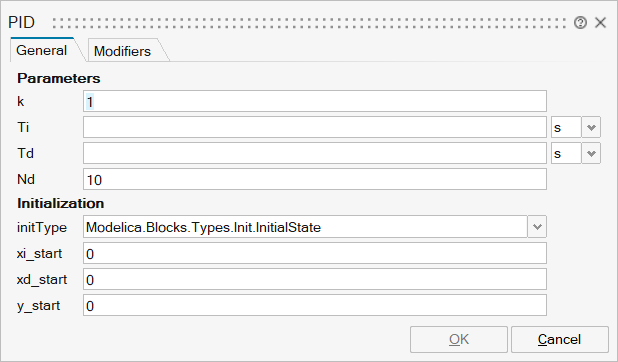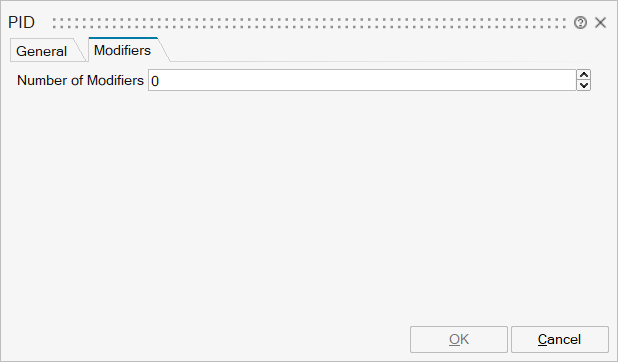# PID## Library

Modelica/Blocks/Continuous

## Description

This is the text-book version of a PID-controller. For a more practically useful PID-controller, use block LimPID.

The PID block can be initialized in different ways controlled by parameter initType. The possible values of initType are defined in Modelica.Blocks.Types.InitPID. This type is identical to Types.Init, with the only exception that the additional option DoNotUse_InitialIntegratorState is added for backward compatibility reasons (= integrator is initialized with InitialState whereas differential part is initialized with NoInit which was the initialization in version 2.2 of the Modelica standard library).

Based on the setting of initType, the integrator (I) and derivative (D) blocks inside the PID controller are initialized according to the following table:

 initType I.initType D.initType NoInit NoInit NoInit SteadyState SteadyState SteadyState InitialState InitialState InitialState InitialOutput and initial equation: y = y_start NoInit SteadyState DoNotUse_InitialIntegratorState InitialState NoInit

In many cases, the most useful initial condition is SteadyState because initial transients are then no longer present. If initType = InitPID.SteadyState, then in some cases difficulties might occur. The reason is the equation of the integrator:

   der(y) = k*u;


The steady state equation "der(x)=0" leads to the condition that the input u to the integrator is zero. If the input u is already (directly or indirectly) defined by another initial condition, then the initialization problem is singular (has none or infinitely many solutions). This situation occurs often for mechanical systems, where, e.g., u = desiredSpeed - measuredSpeed and since speed is both a state and a derivative, it is natural to initialize it with zero. As sketched this is, however, not possible. The solution is to not initialize u or the variable that is used to compute u by an algebraic equation.

## ParametersNameLabelDescriptionData TypeValid Values

mo_k

k

Gain

Scalar

mo_Ti

Ti

Time Constant of Integrator

Scalar

mo_Td

Td

Time Constant of Derivative block

Scalar

mo_Nd

Nd

The higher Nd, the more ideal the derivative block

Scalar

mo_initType

initType

Type of initialization (1: no init, 2: steady state, 3: initial state, 4: initial output)

Structure

mo_initType/choice1

No initialization (start values are used as guess values with fixed=false)

Number

0
1

mo_initType/choice2

Steady state initialization (derivatives of states are zero)

Number

0
1

mo_initType/choice3

Initialization with initial states

Number

0
1

mo_initType/choice4

Initialization with initial outputs (and steady state of the states if possible)

Number

0
1

mo_initType/choice5

Do not use, only for backward compatibility (initialize only integrator state)

Number

0
1

mo_xi_start

xi_start

Initial or guess value for integrator output (= integrator state)

Scalar

mo_xd_start

xd_start

Initial or guess value for state of derivative block

Scalar

mo_y_start

y_start

Initial value of output

Scalar

mo_unitTime

unitTime

ScalarNameLabelDescriptionData TypeValid Values

mo_u

u

u

Structure

mo_u/fixed

fixed

Cell of scalars

true
false

mo_u/start

start

Cell of scalars

mo_y

y

y

Structure

mo_y/fixed

fixed

Cell of scalars

true
false

mo_y/start

start

Cell of scalars

## Ports

NameTypeDescriptionIO TypeNumber

u

implicit

Connector of Real input signal

input

1

y

implicit

Connector of Real output signal

output

1